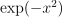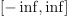/NumPy 1.17

# numpy.polynomial.hermite.hermweight

`numpy.polynomial.hermite.hermweight(x)` [source]

Weight function of the Hermite polynomials.

The weight function isand the interval of integration is. the Hermite polynomials are orthogonal, but not normalized, with respect to this weight function.

Parameters: `x : array_like` Values at which the weight function will be computed. `w : ndarray` The weight function at `x`.

#### Notes

New in version 1.7.0.